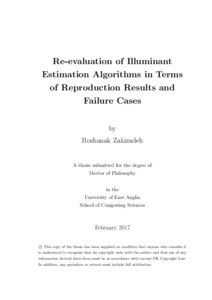# Re-evaluation of illuminant estimation algorithms in terms of reproduction results and failure cases

Zakizadeh, Roshanak (2017) Re-evaluation of illuminant estimation algorithms in terms of reproduction results and failure cases. Doctoral thesis, University of East Anglia.Preview
PDF

## Abstract

Illuminant estimation algorithms are usually evaluated by measuring the recovery
angular error, the angle between the RGB vectors of the estimated and ground-truth
illuminants. However, this metric reports a wide range of errors for an
algorithm-scene pair viewed under multiple lights. In this thesis, a new metric,
“Reproduction Angular Error”, is introduced which is an improvement over the
old metric and enables us to evaluate the performance of the algorithms based
on the reproduced white surface by the estimated illuminant rather than the estimated
illuminant itself. Adopting new reproduction error is shown to both effect
the overall ranking of algorithms as well as the choice of optimal parameters for
particular approaches.
A psychovisual image preference experiment is carried out to investigate whether
human observers prefer colour balanced images predicted by, respectively, the reproduction
or recovery error metric. Human observers rank algorithms mostly
according to the reproduction angular error in comparison with the recovery angular
error.
Whether recovery or reproduction error is used, the common approach to measuring
algorithm performance is to calculate accurate summary statistics over a
dataset. Mean, median and percentile summary errors are often employed. However,
these aggregate statistics, by definition, make it hard to predict performance
for individual images or to discover whether there are certain “hard images” where
some illuminant estimation algorithms commonly fail. Not only do we find that
such hard images exist, based only on the outputs of simple algorithms we provide
an algorithm for identifying these hard images (which can then be assessed usingView Item